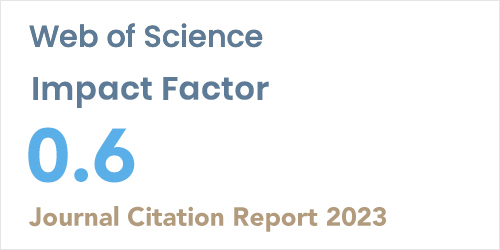ISSN: 1304-7191 | E-ISSN: 1304-7205
Volume: 41 Issue: 4 Year: 2023Computation of fundamental group of a finite hypergroup
1Department of Mathematics, University of Mazandaran, Babolsar-IRAN
2School of Mathematics, University of Tehran, Tehran-IRAN
Sigma J Eng Nat Sci 2018; 9(): 107-125

#### Abstract

Let (H, ) be a hypergroup and * be the fundamental relation on H, that is, the smallest equivalence relation on H such that the quotient (H/*, ) is a group. The purpose of this paper is to compute the fundamental group of a given finite hypergroup. In this regards, first obtain some algebraic results to obtain equivalence classes of the fundamental relation and the we introduce an algorithm to compute these classes. Also, based on these algorithms we develop an application to construct the equivalence classes of * and hence to compute the fundamental group, (H/*, ). Furthermore, we use a sub-program to produce all hypergroups (up to isomorphism) of order less than or equal 3 and obtain their fundamental groups. Finally, we examine the algorithm and program by some examples to compute the fundamental groups of hypergroups of various orders.